#### 期刊菜单

Research on Infrared Image Target Recognition Algorithm Based on Improved YOLOv3
DOI: 10.12677/GST.2022.102007, PDF , HTML, XML, 下载: 243  浏览: 375

Abstract: Aiming at the problem of low accuracy of pedestrian and vehicle target detection in automatic driving at night, this paper proposes an infrared image target recognition algorithm based on improved YOLOv3. First, the algorithm improves the feature extraction ability by increasing the number of convolutions of large-size images in the backbone, and replaces subsequent conventional convolutions with depthwise separable convolutions to reduce the amount of model parameters and improve the running speed; In the scale feature fusion, the feature fusion structure is replaced by the Panet structure to improve the utilization of the underlying information; finally, Distance-IoU (DIoU) is used as the archor loss function to speed up the model convergence. The test results on the Flir image data set show that the improved model of the proposed improved YOLOv3 infrared recognition algorithm achieves better detection accuracy in terms of precision and recall when the model size is almost unchanged. Compared with YOLOv3, there are 2.94% and 3.12% increases in pedestrians and cars, respectively, and the average AP also increases by 3.03%. Experiments show that the improved method not only improves the detection accuracy, but also reduces the amount of models and improves the detection speed.

1. 引言

YOLO算法相较于R-CNN系列算法，仅通过一个卷积网络就能实现对目标物体的位置与类别的检测，凭借其实时性高、便于操作的特点得到了广泛应用。而YOLOv3作为YOLO系列的巅峰之作，不但延续了YOLO系列的便捷性，更是做出了改进。首先在特征提取部分采用darknet-53的backbone代替之前的darknet-19，实现多尺度特征融合，在保证了实用性的同时，又提高了准确性。而在无人驾驶领域对于车辆和行人的目标检测，YOLOv3的灵活性满足了笔者对于目标检测的需要，同时针对于目标检测算法在夜间环境下的检测精度较低的问题，采用Flir红外影像数据集，利用红外影像夜间成像的优势，对于YOLOv3的backbone、特征融合与损失函数三方面进行微调改进，实现对于夜间车辆与行人的目标检测。

2. 改进的YOLOv3目标检测算法

2.1. YOLOv3基本原理

YOLOv3是一阶段(One stage)的目标检测算法，将输入图像划分成三种不同网格，分别对应目标检测的小尺寸目标，中尺寸目标，大尺寸目标。YOLOv3的backbone采用DarkNet-53  的残差神经网络，能够在加深网络的同时又较好地解决梯度消失的问题，有助于数据训练和特征提取融合。YOLOv3的网格结构如图1所示。在多尺度特征融合方面，YOLOv3采用FPN (feature pyramid networks)  特征融合结构。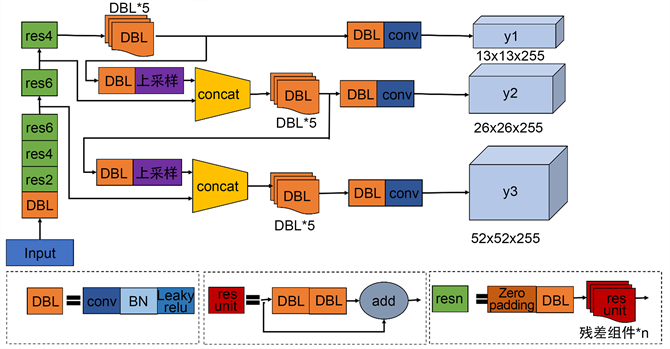Figure 1. YOLOv3 structure diagram

2.2. 深度可分离卷积

2017年Howard  等人提出了深度可分离卷积(depthwise separable convolutions)，通过分步卷积方法大幅降低了模型的参数量。因此为了防止模型参数量过大的问题，本方法将后三个常规卷积更换为深度可分离卷积。深度可分离卷积主要分为两个过程，分别为逐通道卷积DW (Depthwise Convolution)和逐点卷积PW (Pointwise Convolution)。首先，图像输入经过第一次卷积运算，逐通道卷积的一个卷积核负责一个通道，卷积核的数量与上层的通道数一致，计算量为卷积核W × 卷积核H × (图片W − 卷积核W + 1) × (图片H − 卷积核H + 1) × 输入通道数。但逐通道卷积生成的Feature map数量与输入层的通道数相同，没有有效利用不同通道在相同空间位置上的Feature信息，因此需要后续逐点卷积对于生成的Feature map进行组合。逐点卷积部分则是进行1 × 1卷积，进行单点上的特征提取，将上一步生成的Feature map在深度方向上进行加权组合，生成新的Feature map。其计算量为特征层W×特征层H×输入通道数 ×输出通道数。

2.3. 模型改进

2.3.1. Backbone

YOLOv3的backbone采用的是由残差网络(Residual)组成的Darknet-53，在加深网络的同时又能较好地解决梯度消失问题。本文为减少图像在卷积过程中损失过多信息，将前两个残差单元的卷积次数分别从1，2增至2，4，提高大尺寸图像的特征提取能力，改进后的残差网络进行特征提取流程图如图3。其中每个残差单元首尾连接，组成一个大残差边，这样较为有效地减少了传统卷积层在特征提取的过程中存在的信息丢失、损耗的问题，有效地保护了信息的完整性，以两个小残差单元为例，改进的残差单元结构如图2所示。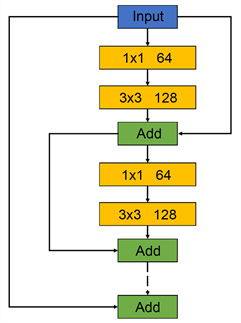Figure 2. Improved residual unit structure diagram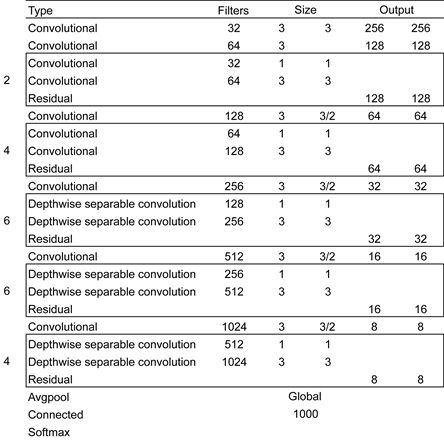Figure 3. Improved residual network feature extraction flowchart

2.3.2. 特征融合

YOLOv3的特征融合结构采用自下而上融合方式，由上层小尺寸特征图经上采样与下层大尺寸特征图融合，然后将融合后的三个尺寸特征图分别检测。而本文采用的是对比度较低的红外影像数据，相比于RGB格式的彩色图像，特征提取更加苦难。所以为了增加网络对提取特征的有效利用，将YOLOv3中的特征融合结构更换为PANet中的结构。PANet结构在FPN的基础上又增加了一个从低层特征层到高层特征层的路径，将低层特征语义信息二次向上层传递，增加了底层特征语义信息的传递率与利用率，结构如图4所示。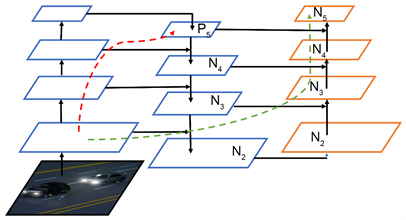Figure 4. Improved feature fusion structure diagram

2.3.3. 损失函数优化

YOLOv3的损失函数由检测物体中心点的坐标误差(x, y)，anchor box的宽高坐标误差(w, h)，置信度误差(confidence)，分类误差(class)组成。具体函数表示如下：

$\begin{array}{c}Loss=-{\lambda }_{coord}\underset{i=0}{\overset{{S}^{2}}{\sum }}\underset{j=0}{\overset{B}{\sum }}{I}_{ij}^{obj}\left[{\stackrel{^}{x}}_{i}^{j}\mathrm{log}\left({x}_{i}^{j}\right)+\left(1-{\stackrel{^}{x}}_{i}^{j}\right)\mathrm{log}\left(1-{x}_{i}^{j}\right)+{\stackrel{^}{y}}_{i}^{j}\mathrm{log}\left({y}_{i}^{j}\right)+\left(1-{\stackrel{^}{y}}_{i}^{j}\right)\mathrm{log}\left(1-{y}_{i}^{j}\right)\right]\\ \text{\hspace{0.17em}}\text{ }\text{ }+{\lambda }_{coord}\frac{1}{2}\underset{i=0}{\overset{{S}^{2}}{\sum }}\underset{j=0}{\overset{B}{\sum }}{I}_{ij}^{obj}\left[{\left({w}_{i}^{j}-{\stackrel{^}{w}}_{i}^{j}\right)}^{2}+{\left({h}_{i}^{j}-{\stackrel{^}{h}}_{i}^{j}\right)}^{2}\right]\\ \text{\hspace{0.17em}}\text{ }\text{ }-\underset{i=0}{\overset{{S}^{2}}{\sum }}\underset{j=0}{\overset{B}{\sum }}{I}_{ij}^{obj}\left[{\stackrel{^}{C}}_{i}^{j}\mathrm{log}\left({C}_{i}^{j}\right)+\left(1-{\stackrel{^}{C}}_{i}^{j}\right)\mathrm{log}\left(1-{C}_{i}^{j}\right)\right]\\ \text{\hspace{0.17em}}\text{ }\text{ }-{\lambda }_{noobj}\underset{i=0}{\overset{{S}^{2}}{\sum }}\underset{j=0}{\overset{B}{\sum }}{I}_{ij}^{noobj}\left[{\stackrel{^}{C}}_{i}^{j}\mathrm{log}\left({C}_{i}^{j}\right)+\left(1-{\stackrel{^}{C}}_{i}^{j}\right)\mathrm{log}\left(1-{C}_{i}^{j}\right)\right]\\ \text{\hspace{0.17em}}\text{ }\text{ }-\underset{i=0}{\overset{{S}^{2}}{\sum }}\underset{j=0}{\overset{B}{\sum }}{I}_{ij}^{obj}\underset{c\in classes}{\sum }\left[{\stackrel{^}{P}}_{i}^{j}\mathrm{log}\left({P}_{i}^{j}\right)+\left(1-{\stackrel{^}{P}}_{i}^{j}\right)\mathrm{log}\left(1-{P}_{i}^{j}\right)\right]\end{array}$

$-{\lambda }_{coord}\underset{i=0}{\overset{{S}^{2}}{\sum }}\underset{j=0}{\overset{B}{\sum }}{I}_{ij}^{obj}\left[{\stackrel{^}{x}}_{i}^{j}\mathrm{log}\left({x}_{i}^{j}\right)+\left(1-{\stackrel{^}{x}}_{i}^{j}\right)\mathrm{log}\left(1-{x}_{i}^{j}\right)+{\stackrel{^}{y}}_{i}^{j}\mathrm{log}\left({y}_{i}^{j}\right)+\left(1-{\stackrel{^}{y}}_{i}^{j}\right)\mathrm{log}\left(1-{y}_{i}^{j}\right)\right]$ 为预测框中心坐标误差，其中 $\left({x}_{i}^{j},{y}_{i}^{j}\right)$ 是YOLOv3的预测框中心点坐标， $\left({\stackrel{^}{x}}_{i}^{j},{\stackrel{^}{y}}_{i}^{j}\right)$ 为设定预测框的中心点坐标， ${I}_{ij}^{obj}$ 为预测框

${\lambda }_{coord}\frac{1}{2}\underset{i=0}{\overset{{S}^{2}}{\sum }}\underset{j=0}{\overset{B}{\sum }}{I}_{ij}^{obj}\left[{\left({w}_{i}^{j}-{\stackrel{^}{w}}_{i}^{j}\right)}^{2}+{\left({h}_{i}^{j}-{\stackrel{^}{h}}_{i}^{j}\right)}^{2}\right]$ 为预测框宽高误差，其中 $\left({w}_{i}^{j},{h}_{i}^{j}\right)$ 为预测框宽高， $\left({\stackrel{^}{w}}_{i}^{j},{\stackrel{^}{h}}_{i}^{j}\right)$

$-\underset{i=0}{\overset{{S}^{2}}{\sum }}\underset{j=0}{\overset{B}{\sum }}{I}_{ij}^{obj}\left[{\stackrel{^}{C}}_{i}^{j}\mathrm{log}\left({C}_{i}^{j}\right)+\left(1-{\stackrel{^}{C}}_{i}^{j}\right)\mathrm{log}\left(1-{C}_{i}^{j}\right)\right]$ 为预测框置信度损失， ${C}_{i}^{j}$ 为预测框中是否含有目标检测物体的概率， ${\stackrel{^}{C}}_{i}^{j}$ 为其真实值。

$-\underset{i=0}{\overset{{S}^{2}}{\sum }}\underset{j=0}{\overset{B}{\sum }}{I}_{ij}^{obj}\underset{c\in classes}{\sum }\left[{\stackrel{^}{P}}_{i}^{j}\mathrm{log}\left({P}_{i}^{j}\right)+\left(1-{\stackrel{^}{P}}_{i}^{j}\right)\mathrm{log}\left(1-{P}_{i}^{j}\right)\right]$ 为预测框中目标物体的类别损失， ${P}_{i}^{j}$ 表示预测框中目标检测物体是否属于检测类别的概率， ${\stackrel{^}{P}}_{i}^{j}$ 其所属类别的真实值。

${\mathcal{L}}_{\text{DIoU}}=1-\text{IoU}+\frac{{\rho }^{2}\left(b,{b}_{gt}\right)}{{c}^{2}}$

3. 实验结果分析与讨论Table 1. Comparative experimental results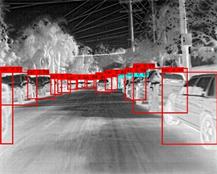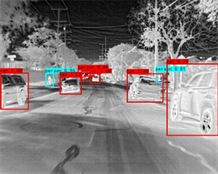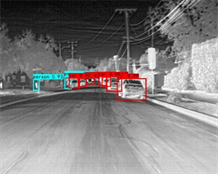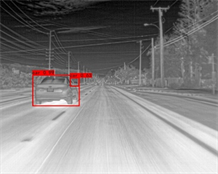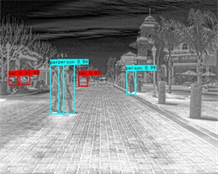Figure 5. Recognition renderings

4. 结论

  Dalal, N. and Triggs, B. (2005) Histograms of Oriented Gradients for Human Detection. IEEE Computer Society Conference on Computer Vision & Pattern Recognition, San Diego, 20-25 June 2005, 886-893.  Ojala, T., Pietikäinen, M. and Harwood, D. (1996) A Comparative Study of Texture Measures with Classification Based on Feature Distributions. Pattern Recognition, 29, 51-59. https://doi.org/10.1016/0031-3203(95)00067-4  Lowe, D.G. (2004) Distinctive Image Features from Scale-Invariant Keypoints. International Journal of Computer Vision, 60, 91-110. https://doi.org/10.1023/B:VISI.0000029664.99615.94  Wren, C.R., Azarbayejani, A.J., Darrell, T.J., et al. (1996) Pfinder: Real-Time Tracking of the Human Body. Proceedings of the Second International Conference on Automatic Face and Gesture Recognition, Killington, 14-16 October 1996, 51-59.  Yin, J., Lei, L., He, L., et al. (2016) The Infrared Moving Object Detection and Security Detection Related Algorithms Based on W4 and Frame Difference. Infrared Physics & Technology, 77, 302-315. https://doi.org/10.1016/j.infrared.2016.06.004  Horn, B.K.P. and Schunck, B.G. (1981) Determining Optical Flow. Artificial Intelligence, 17, 185-203. https://doi.org/10.1016/0004-3702(81)90024-2  Girshick, R., Donahue, J., Darrell, T., et al. (2014) Rich Feature Hierarchies for Accurate Object Detection and Semantic Segmentation. 2014 IEEE Conference on Computer Vision and Pattern Recognition, Columbus, 23-28 June 2014, 580-587. https://doi.org/10.1109/CVPR.2014.81  He, K., Zhang, X., Ren, S., et al. (2014) Spatial Pyramid Pooling in Deep Convolutional Networks for Visual Recognition. IEEE Transactions on Pattern Analysis & Machine Intelligence, 37, 1904-1916. https://doi.org/10.1109/TPAMI.2015.2389824  Girshick, R. (2015) Fast R-CNN. 2015 IEEE International Conference on Computer Vision (ICCV), Santiago, 7-13 December 2015, 1440-1448. https://doi.org/10.1109/ICCV.2015.169  Ren, S., He, K., Girshick, R., et al. (2017) Faster R-CNN: Towards Real-Time Object Detection with Region Proposal Networks. IEEE Transactions on Pattern Analysis and Machine Intelligence, 39, 1137-1149.  Dai, J., Li, Y., He, K., et al. (2016) R-FCN: Object Detection via Region-Based Fully Convolutional Networks. Proceedings of the 30th International Conference on Neural Information Processing Systems, Barcelona, 379.  Sermanet, P., Eigen, D., Zhang, X., et al. (2013) OverFeat: Integrated Recognition, Localization and Detection Using Convolutional Networks. arXiv:1312.6229.  Redmon, J., Divvala, S., Girshick, R., et al. (2016) You Only Look Once: Unified, Real-Time Object Detection. 2016 IEEE Conference on Computer Vision and Pattern Recognition (CVPR), Las Vegas, 27-30 June 2016, 779-788. https://doi.org/10.1109/CVPR.2016.91  Redmon, J. and Farhadi, A. (2017) YOLO9000: Better, Faster, Stronger. 2017 IEEE Conference on Computer Vision and Pattern Recognition (CVPR), Honolulu, 21-26 July 2017, 6517-6525. https://doi.org/10.1109/CVPR.2017.690  Redmon, J. and Farhadi, A. (2018) YOLOv3: An Incremental Improvement. arXiv: 1804.02767..  Liu, W., Anguelov, D., Erhan, D., et al. (2016) SSD: Single Shot MultiBox Detector. Springer, Cham.  Lin, T.Y., Goyal, P., Girshick, R., et al. (2017) Focal Loss for Dense Object Detection. 2017 IEEE International Conference on Computer Vision (ICCV), Venice, 22-29 October 2017, 2999-3007. https://doi.org/10.1109/ICCV.2017.324  Lin, T.Y., Dollar, P., Girshick, R., et al. (2017) Feature Pyramid Networks for Object Detection. 2017 IEEE Conference on Computer Vision and Pattern Recognition (CVPR), Honolulu, 21-26 July 2017, 936-944. https://doi.org/10.1109/CVPR.2017.106  Liu, S., Qi, L., Qin, H., et al. (2018) Path Aggregation Network for Instance Segmentation. 2018 IEEE/CVF Conference on Computer Vision and Pattern Recognition, Salt Lake City, 18-23 June 2018, 8759-8768. https://doi.org/10.1109/CVPR.2018.00913  Howard, A.G., Zhu, M., Chen, B., et al. (2017) MobileNets: Efficient Convolutional Neural Networks for Mobile Vision Applications. arXiv:1704.04861.  Zheng, Z., Wang, P., Ren, D., et al. (2020) Enhancing Geometric Factors in Model Learning and Inference for Object Detection and Instance Segmentation. IEEE Transactions on Cybernetics. https://doi.org/10.1109/TCYB.2021.3095305  Zheng, Z., Wang, P., Liu, W., et al. (2020) Distance-IoU Loss: Faster and Better Learning for Bounding Box Regression. Proceedings of the AAAI Conference on Artificial Intelligence, 34, 12993-13000.